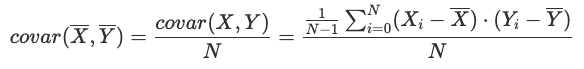# Standard Error

The standard error of the means is required for computing the confidence interval and p-value of a metric delta. It can be obtained by dividing the sample standard deviation of X by the number of users in the group.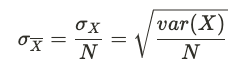Note that standard deviation is the square root of the variance. Since variances are easier to manipulate algebraically, here we derive the variance for each metric type and then take the square root to obatin the confidence intervals.

## Computing Variance​

The variance of the absolute metric delta is simply the sum of the variances of the test and control means: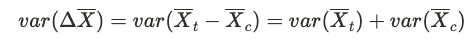In other words, it comes down to correclty calcualting the variance of the means for each group.

### Count and Sum Metrics​

For count and sum metrics, the variance of the sample mean for a given group is obtained directly from the sample variance: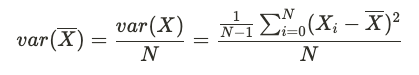Where:

• N is the number of users in the group
• Xi is the metric value for user i
• X-bar is the user-level average of X for users in that group

### Ratio and Mean Metrics​

The variance of ratio and mean metrics depends upon the numberator and denominator variables, which are typically correlated. For example, consider a clicks per session metric. The number of clicks and the number of sessions are two sets of obervations coming from the same group of users, so they are not independent from each other. To properly account for this correlation, the variance of the mean of a ratio metric R is obtained using the delta method: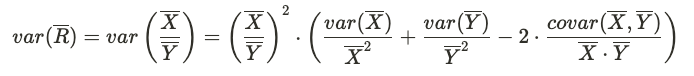where the variance of the numerator and denominator means are computed in the same way as detailed above for count metrics, and the covariance is